# Pimpri Chinchwad polytechnics COURSE FLUID MECHANICS AND MACHINERY

• Slides: 24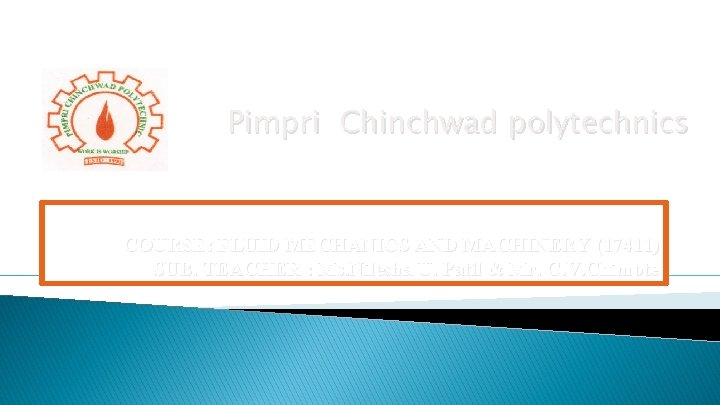Pimpri Chinchwad polytechnics COURSE: FLUID MECHANICS AND MACHINERY (17411) SUB. TEACHER : Ms. Nilesha U. Patil & Mr. C. V. Chimote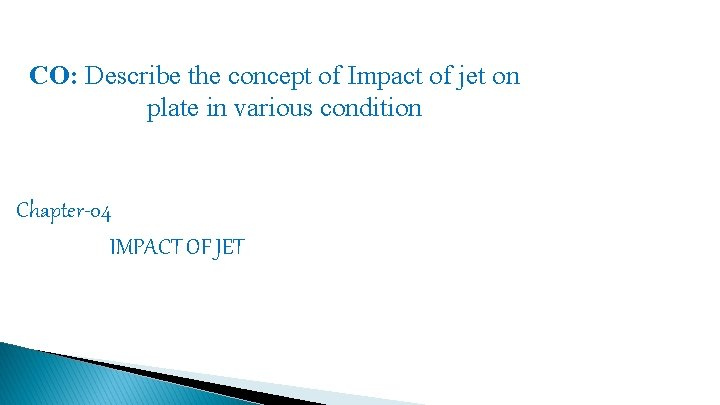CO: Describe the concept of Impact of jet on plate in various condition Chapter-04 IMPACT OF JET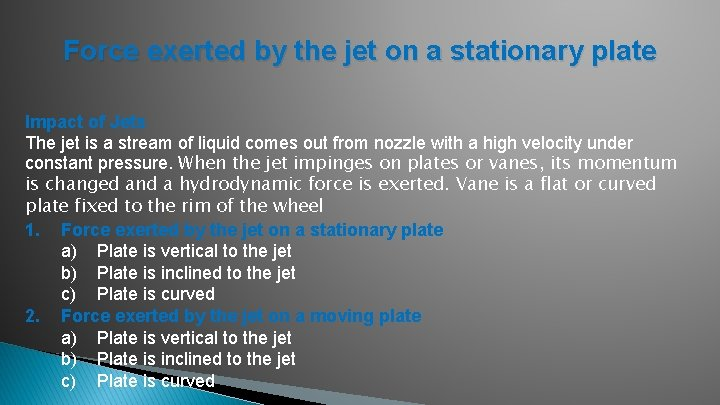Force exerted by the jet on a stationary plate Impact of Jets The jet is a stream of liquid comes out from nozzle with a high velocity under constant pressure. When the jet impinges on plates or vanes, its momentum is changed and a hydrodynamic force is exerted. Vane is a flat or curved plate fixed to the rim of the wheel 1. Force exerted by the jet on a stationary plate a) Plate is vertical to the jet b) Plate is inclined to the jet c) Plate is curved 2. Force exerted by the jet on a moving plate a) Plate is vertical to the jet b) Plate is inclined to the jet c) Plate is curvedImpulse-Momentum Principle From Newton's 2 nd Law: F = m a = m (V 1 - V 2) / t Impulse of a force is given by the change in momentum caused by the force on the body. Ft = m. V 1 – m. V 2 = Initial Momentum – Final Momentum Force exerted by jet on the plate in the direction of jet, F = m (V 1 – V 2) / t = (Mass / Time) (Initial Velocity – Final Velocity) = (ρQ) (V 1 – V 2) = (ρa. V) (V 1 – V 2)Force exerted by the jet on a stationary plate Plate is vertical to the jet F= 2 a. V F= 2 a(V-U) If Plate is moving at a velocity of ‘U’ m/s,Problems: 1. A jet of water 50 mm diameter strikes a flat plate held normal to the direction of jet. Estimate the force exerted and work done by the jet if a. The plate is stationary b. The plate is moving with a velocity of 1 m/s away from the jet along the line of jet. The discharge through the nozzle is 76 lps. 2. A jet of water 50 mm diameter exerts a force of 3 k. N on a flat vane held perpendicular to the direction of jet. Find the mass flow rate.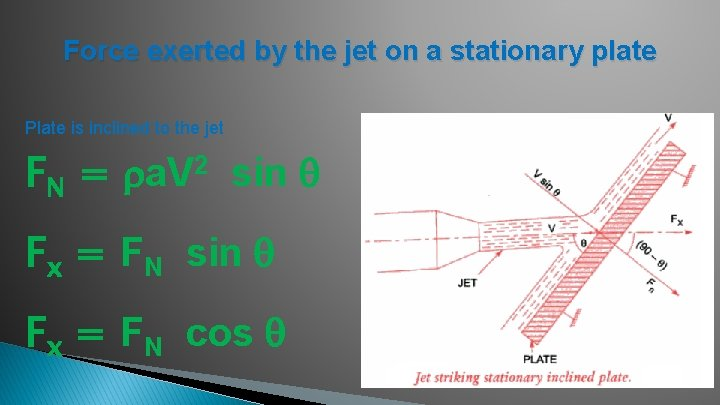Force exerted by the jet on a stationary plate Plate is inclined to the jet FN = a. V 2 sin Fx = FN cos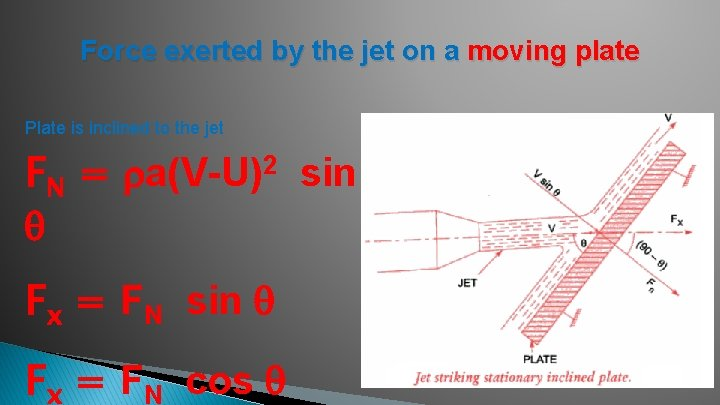Force exerted by the jet on a moving plate Plate is inclined to the jet FN = a(V-U)2 sin Fx = FN cos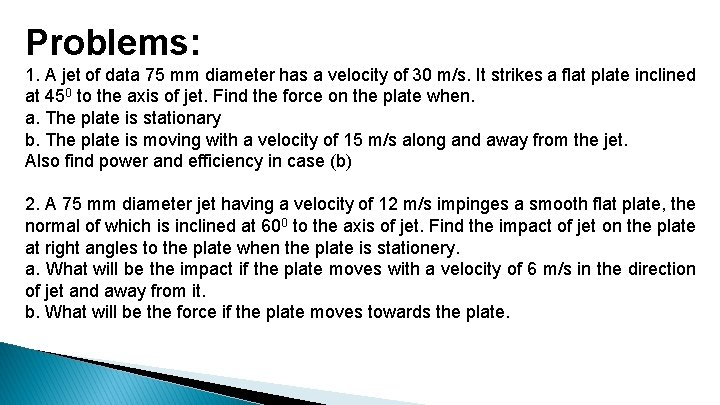Problems: 1. A jet of data 75 mm diameter has a velocity of 30 m/s. It strikes a flat plate inclined at 450 to the axis of jet. Find the force on the plate when. a. The plate is stationary b. The plate is moving with a velocity of 15 m/s along and away from the jet. Also find power and efficiency in case (b) 2. A 75 mm diameter jet having a velocity of 12 m/s impinges a smooth flat plate, the normal of which is inclined at 600 to the axis of jet. Find the impact of jet on the plate at right angles to the plate when the plate is stationery. a. What will be the impact if the plate moves with a velocity of 6 m/s in the direction of jet and away from it. b. What will be the force if the plate moves towards the plate.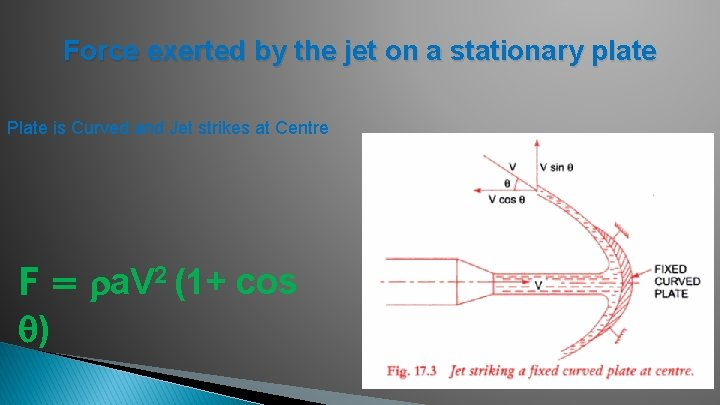Force exerted by the jet on a stationary plate Plate is Curved and Jet strikes at Centre F = a. V 2 (1+ cos )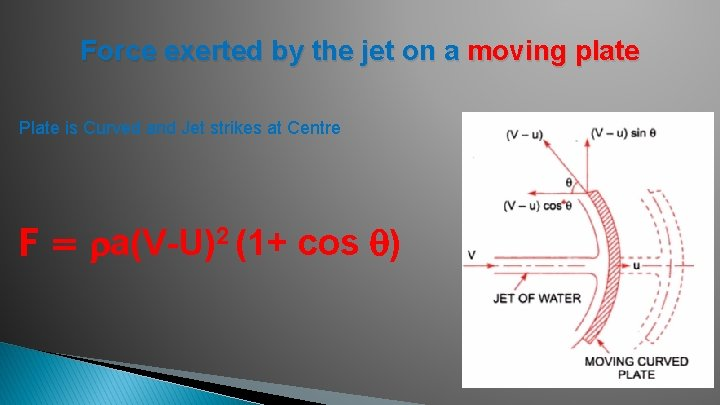Force exerted by the jet on a moving plate Plate is Curved and Jet strikes at Centre F = a(V-U)2 (1+ cos )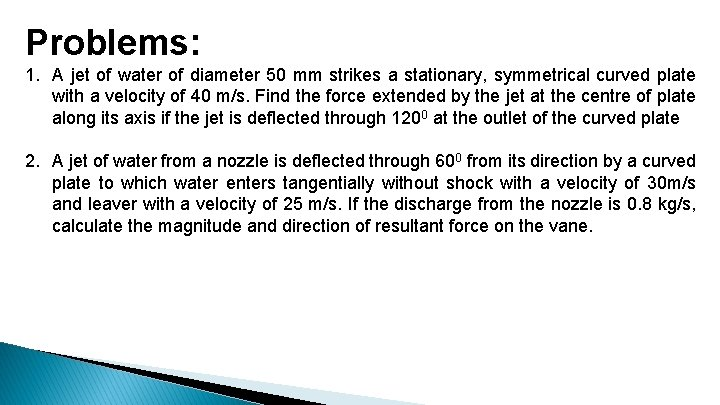Problems: 1. A jet of water of diameter 50 mm strikes a stationary, symmetrical curved plate with a velocity of 40 m/s. Find the force extended by the jet at the centre of plate along its axis if the jet is deflected through 1200 at the outlet of the curved plate 2. A jet of water from a nozzle is deflected through 600 from its direction by a curved plate to which water enters tangentially without shock with a velocity of 30 m/s and leaver with a velocity of 25 m/s. If the discharge from the nozzle is 0. 8 kg/s, calculate the magnitude and direction of resultant force on the vane.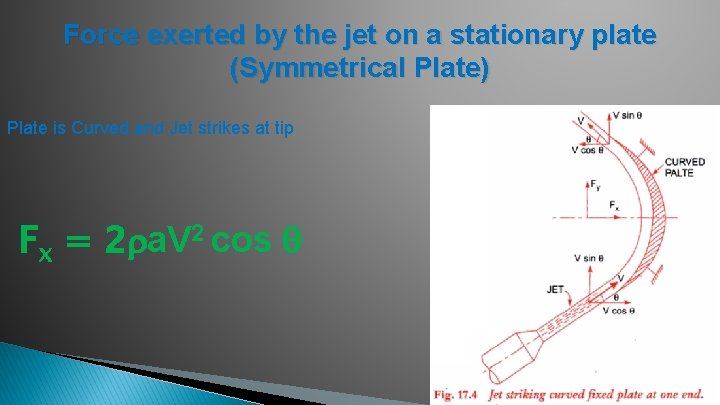Force exerted by the jet on a stationary plate (Symmetrical Plate) Plate is Curved and Jet strikes at tip Fx = 2 a. V 2 cos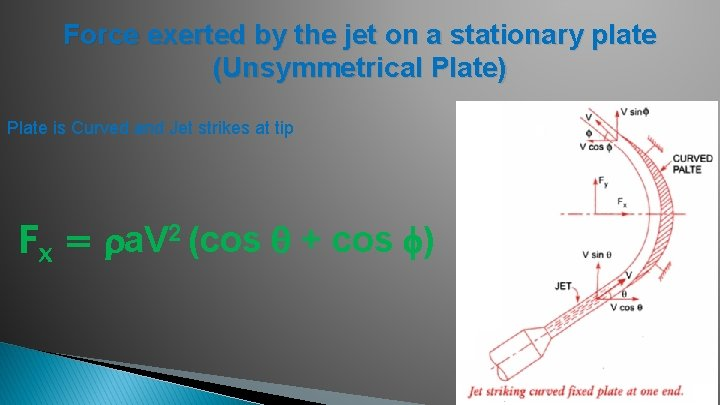Force exerted by the jet on a stationary plate (Unsymmetrical Plate) Plate is Curved and Jet strikes at tip Fx = a. V 2 (cos + cos )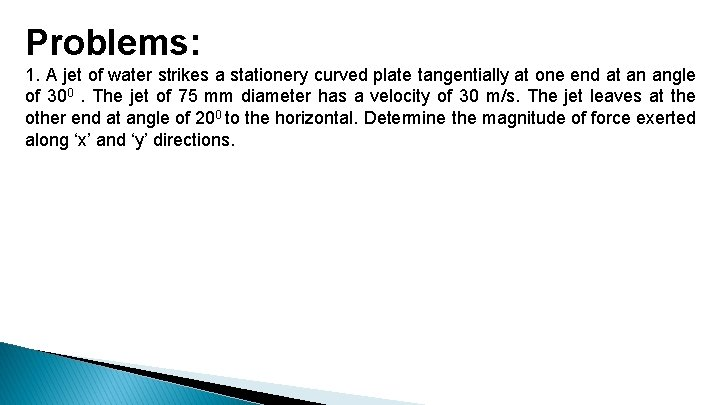Problems: 1. A jet of water strikes a stationery curved plate tangentially at one end at an angle of 300. The jet of 75 mm diameter has a velocity of 30 m/s. The jet leaves at the other end at angle of 200 to the horizontal. Determine the magnitude of force exerted along ‘x’ and ‘y’ directions.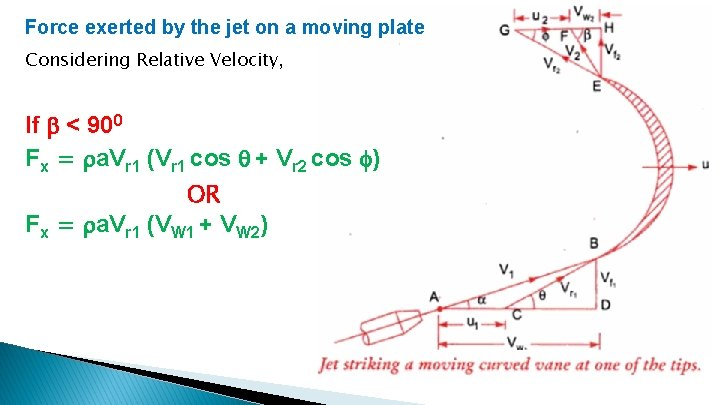Force exerted by the jet on a moving plate Considering Relative Velocity, If < 900 Fx = a. Vr 1 (Vr 1 cos + Vr 2 cos ) OR Fx = a. Vr 1 (VW 1 + VW 2)Force exerted by the jet on a moving plate Considering Relative Velocity, If = 900 Fx = a. Vr 1 (Vr 1 cos – Vr 2 cos ) OR Fx = a. Vr 1 (VW 1)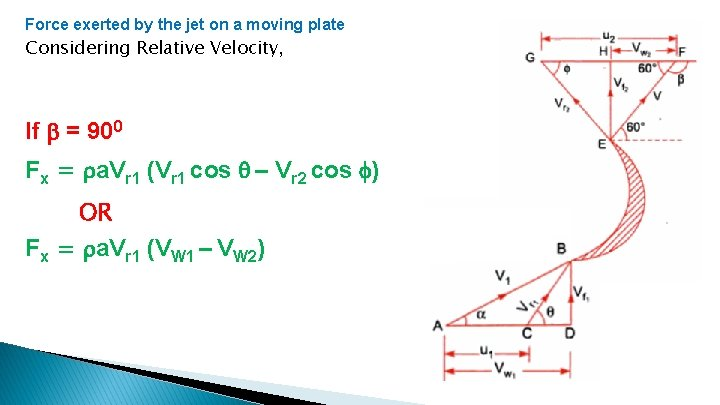Force exerted by the jet on a moving plate Considering Relative Velocity, If = 900 Fx = a. Vr 1 (Vr 1 cos – Vr 2 cos ) OR Fx = a. Vr 1 (VW 1 – VW 2)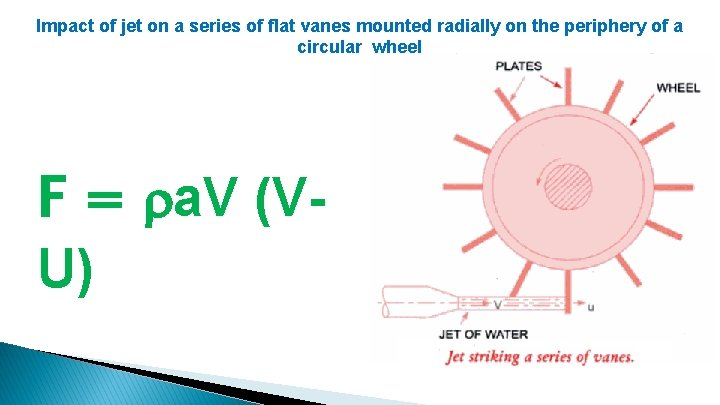Impact of jet on a series of flat vanes mounted radially on the periphery of a circular wheel F = a. V (VU)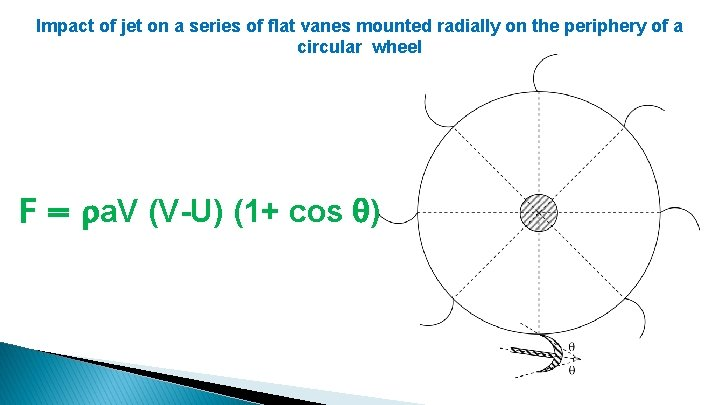Impact of jet on a series of flat vanes mounted radially on the periphery of a circular wheel F = a. V (V-U) (1+ cos )Problems: 1. A jet of water of diameter 75 mm strikes a curved plate at its centre with a velocity of 25 m/s. The curved plate is moving with a velocity of 10 m/s along the direction of jet. If the jet gets deflected through 1650 in the smooth vane, compute. a) Force exerted by the jet. b) Power of jet. c) Efficiency of jet. 2. A jet of water impinges a curved plate with a velocity of 20 m/s making an angle of 200 with the direction of motion of vane at inlet and leaves at 1300 to the direction of motion at outlet. The vane is moving with a velocity of 10 m/s. Compute. i) Vane angles, so that water enters and leaves without shock. ii) Work done per unit mass flow rate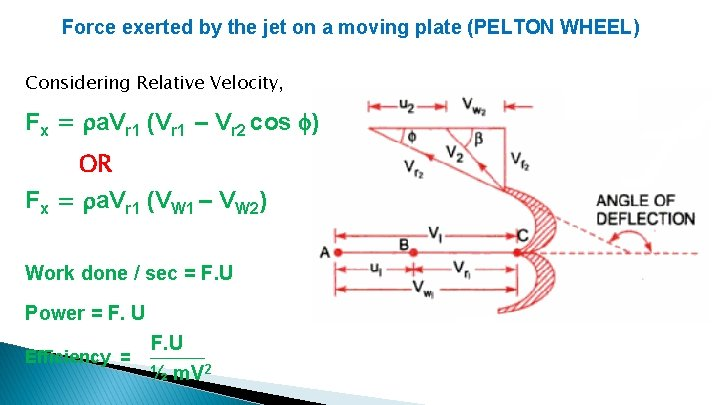Force exerted by the jet on a moving plate (PELTON WHEEL) Considering Relative Velocity, Fx = a. Vr 1 (Vr 1 – Vr 2 cos ) OR Fx = a. Vr 1 (VW 1 – VW 2) Work done / sec = F. U Power = F. U Efficiency = F. U ½ m. V 2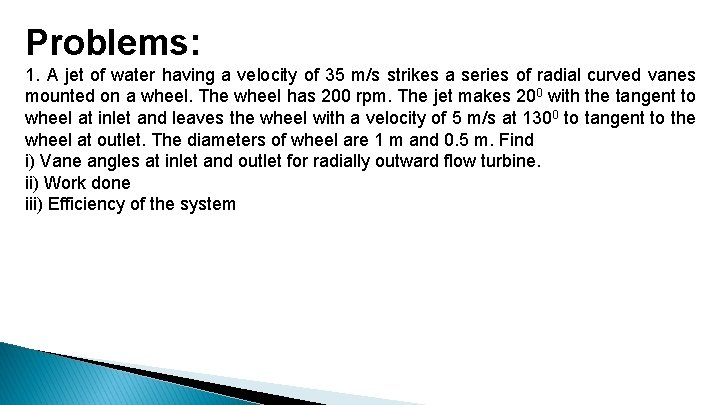Problems: 1. A jet of water having a velocity of 35 m/s strikes a series of radial curved vanes mounted on a wheel. The wheel has 200 rpm. The jet makes 200 with the tangent to wheel at inlet and leaves the wheel with a velocity of 5 m/s at 1300 to tangent to the wheel at outlet. The diameters of wheel are 1 m and 0. 5 m. Find i) Vane angles at inlet and outlet for radially outward flow turbine. ii) Work done iii) Efficiency of the systemTHANK YOU